• Post Author:

#### EMF (electromotive force) of a cell

The maximum potential difference that exists between two electrodes of a cell is called the electromotive force (EMF) of the cell.

In other words, electromotive force is difference in potential which causes the current to flow from an electrode of higher potential to an electrode of a lower potential.

• It is also known as cell potential
• It is measured in volts.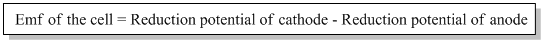The emf of a cell measured under standard conditions is called standard emf. It is denoted by ‘E0cell’. The standard conditions are:

1. Concentration of electrolytic solution is 1M.
2. Temperature of the system is 250C.
3. Pressure of the gas is 1 atmosphere.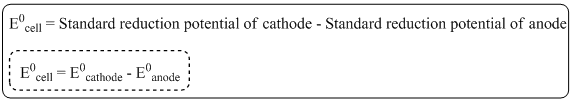Q) A galvanic cell is represented as

Zn/Zn++//Cd++/Cd

Write the ell reaction and calculate the standard emf of the cell.

Given that E0Zn++/Zn = -0.70V and E0Cd++/Cd = -0.40V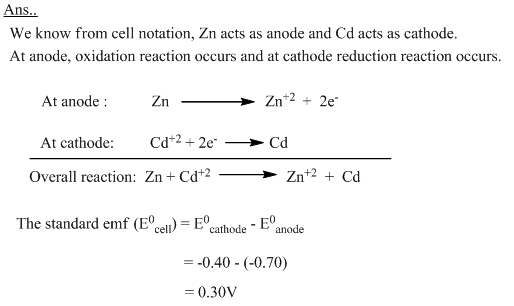#### Nernst equation

The Nernst equation provides the relation between the cell potential of an electrochemical cell, the standard cell potential, temperature and the equilibrium constant.

Mathematically, the Nernst equation can be expressed as: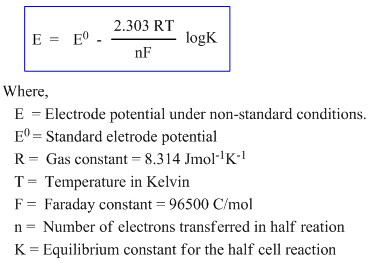For an oxidation half-cell reaction, when the metal electrode M gives Mn+ ion,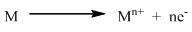The Nernst equation takes the form: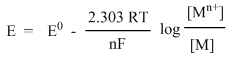The concentration of solid metal [M] is equal to zero. Hence,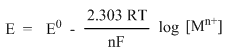At 250C:

On putting the values of R, F and T at 250C, the quantity 2.303RT/F comes to be 0.0591.

Thus, at 250C, the Nernst equation can be written as: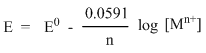• This equation is for half cell in which oxidation occurs.
• In case of reduction half reaction, the sign of E is reversed.
• If the Nernst equation is applied to the complete cell as a whole, then it will be in the form: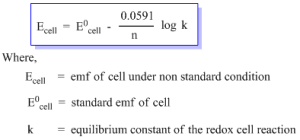Q) Calculate the emf of the cell.

Zn/Zn++(0.001M)//Ag+(0.1M)/Ag

The standard electrode potential E0 of Ag/Ag+ is 0.80V and Zn/Zn++ is -0.76V.

Ans….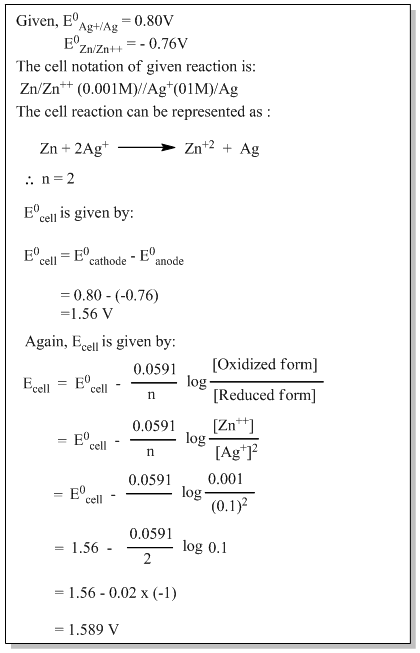#### Nernst equation applications

Nernst equation can be used to calculate the following:

• Single electrode potential (oxidation or reduction potential) at any conditions.
• Standard electrode potential.
• Comparing the relative ability as an oxidizing or reducing agent.
• Emf of an electrochemical cell
• Unknown ionic concentrations.
• pH of a solution also can be measured using Nernst equation.